# Passive High Pass Filter

Published  February 21, 2018   0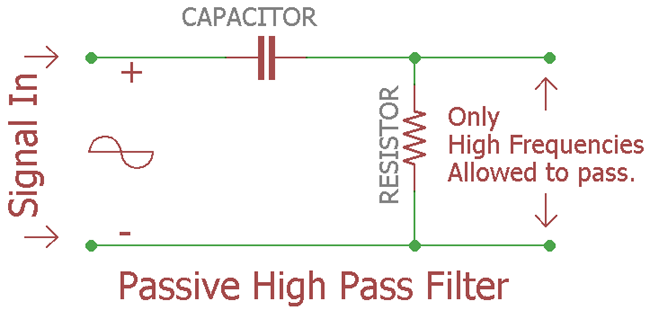Previously we discussed Passive Low Pass Filter, now it is the time to look insight of passive high pass filter.

Same as like before, if you look into the name it shows “Passive”, “High”, “Pass” and “Filter”. So, as the name suggests, it is a filter that will block Low frequencies, but pass the high frequency above the predetermined value, which will be calculated by the formula.

It is “passive” which means no external power, no amplification of the input signal; we will make the circuit using “passive” components which do not requires any external power source. The passive components are same as Low pass filter but the connection order will be exactly reversed. The passive components are Resistor (R) and
Capacitor (C). Again it is a RC filter configuration.

Let’s see what happens if we construct the circuit and check the response or “Bode Plot”…

Here is the circuit in this image: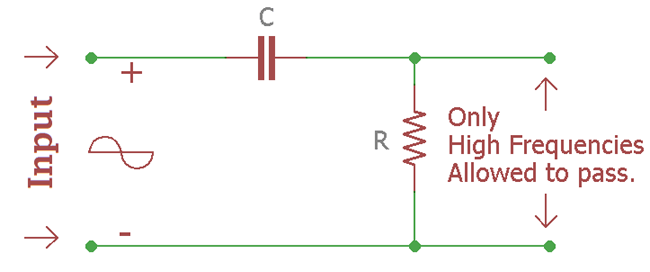This is a RC filter. Generally an input signal is applied to this series combination of non-polarized capacitor and resistor. It is a first order filter as there is only one reactive component in the circuitry that is capacitor. The filtered output will be available across resistor. The combination of this duo are exactly opposite of low pass filter. If we compare the circuit with the low pass filter we will see that the position of resistor and capacitor is interchanged.

### How High Pass filter works?

At low frequencies the reactance of the capacitor will be very large that it will act like an open circuit and block the input signal below the cut-off frequency point (fc). But when the cut-off frequency point reached the reactance of the capacitor will start to reduce and allow the signal to pass directly. We will see this in detail in the frequency response curve.

Here is the curve how it’s look alike at the output of the capacitor:-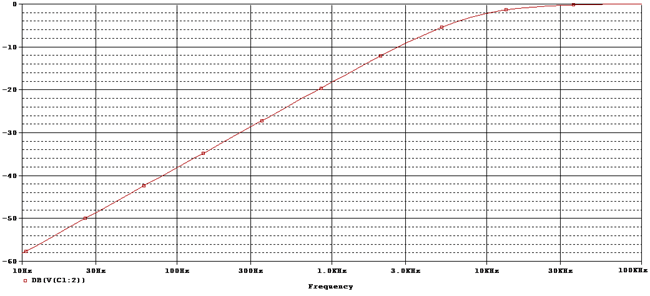### Frequency Response and Cut-Off Frequency

This is the frequency response curve of that first order High pass filter circuit.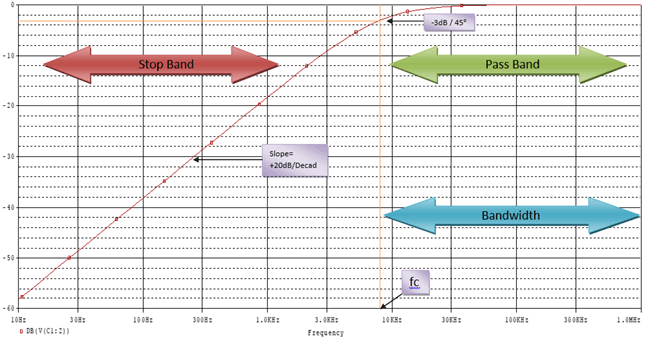fc Is the cutoff frequency of the filter. At -3dB point the signal is allowed to pass. This -3dB also denotes the cutoff frequency. From 10Hz to the cut-off frequency the signal is not allowed to pass as the frequency is Low frequency, at this point it is the stop band portion where the signal is not allowed to pass from the filter but above the cut-off frequency after -3dB the portion is called as pass band position where the signal is allowed to pass. The slope of the curve is +20dB per decade. Exactly opposite of Low pass filter.

The formula of Calculating gain is same as we used in our previous tutorial in passive Low pass filter.

`Gain(dB) = 20 log (Vout / Vin)`

After the cut-off signal the responses of the circuit gradually increase to Vin from 0 and this increment happens at a rate of +20dB/Decade. If we calculate the increase per octave it will be 6dB.

This Frequency Response Curve is the Bode Plot of High pass filter. By selecting proper capacitor and proper resistor we could stop Low frequencies, limit signal passing through the filter circuitry without affecting the signal as there is no active response.

In the above image, there is a word Bandwidth. It’s signifying after what frequency the signal will allow to pass. So, if it is a 600 Khz High pass filter then the bandwidth will be from 600Khz to Infinity. As it will allow to pass all signals above the cut-off frequency.

At the cut-off frequency we will get -3dB gain. At that point if we compare output signal amplitude with input signal then we will see that the output signal amplitude would be 70.7% of the input signal. Also in -3dB gain the capacitive reactance and resistance would be equal. R= Xc.

### What is the formula of Cut-off Frequency?

The formula of Cut-off frequency is exactly same as like Low Pass filter.

`fc = 1 / 2πRC`

So, R is resistance and C is capacitance. If we put the value we will know the cutoff frequency.

### Output Voltage Calculation

Let’s see the first image, the circuitry where 1 resistor and one capacitor are used to form a High pass filter or RC circuit.

When DC signal applied across the circuit it’s resistance of the circuit which creates drop when current is flowing. But in case of an AC signal it’s not resistance but impedance is responsible for voltage drop, which measured in Ohms too.

In the RC circuit there are two resistive things. One is resistance and other one is the capacitive reactance of the capacitor. So, we need to measure the capacitive reactance of the capacitor first as it will needed to calculating impedance of the circuitry.

First resistive opposition is capacitive reactance, the formula is:-

`Xc = 1 / 2πfC`

The output of the formula will be in Ohms, as Ohms is the unit of capacitive reactance because it is an opposition means Resistance.

The Second opposition is the resistor itself. Value of resistor is also a resistance.

So, combining this two opposition we will get the total resistance, which is impedance in RC (AC signal input) circuit.

Impedance denotes as Z

The Formula is:-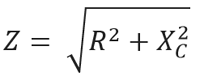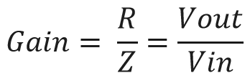As discussed before in the low frequency the reactance of the capacitor is too high that it is act as an open circuit, the reactance of the capacitor is Infinity at low frequency so it is block the signal. Output gain is 0 at that time, and due to the block the output voltage remains 0 until cut-off frequency is reached.

But in High frequency the opposite will happen the reactance of the capacitor is too low that it act as an short circuit, the reactance of the capacitor is 0 at high frequency so it is pass the signal. Output gain is 1 at that time, that is Unity gain situation and due to unity gain the output voltage is same as the input voltage after the cut-off frequency is reached.

### Example with Calculation

As we already know what actually happening inside the circuit and How to find out the value. Let’s choose practical values.

Let’s pick up most common value in resistor and capacitor, 330k and 100pF. We selected the value as it is widely available and it is easier to calculate.

Let’s see what will be the cut-off frequency and what will be the Output voltage.

Cut off Frequency will be:-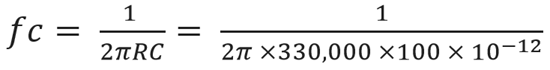By solving this equation the cut-off frequency is 4825Hz or 4.825Khz.

Let’s See whether it is true or not…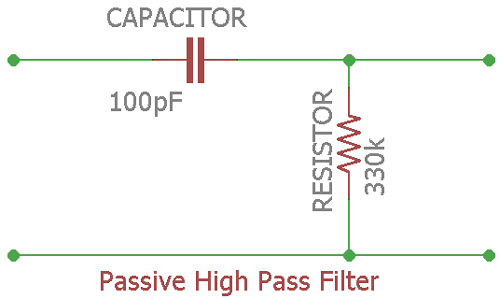This is the circuit of the example.

As the frequency response described before that at the cut-off frequency the dB will be
-3dB, Irrespective of the frequencies. We will search -3dB at the output signal and see whether it is 4825Hz (4.825Khz) or not.

Here is the frequency response:-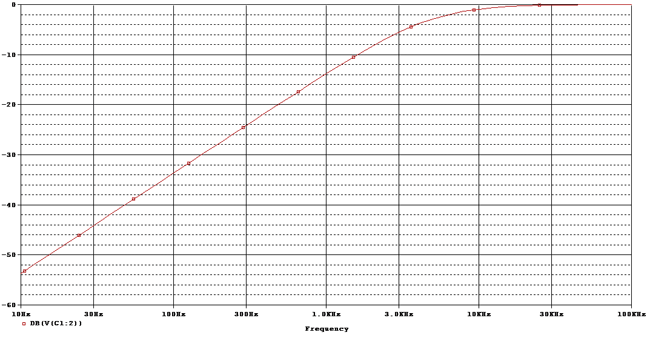Let’s set the cursor at -3dB and see the result.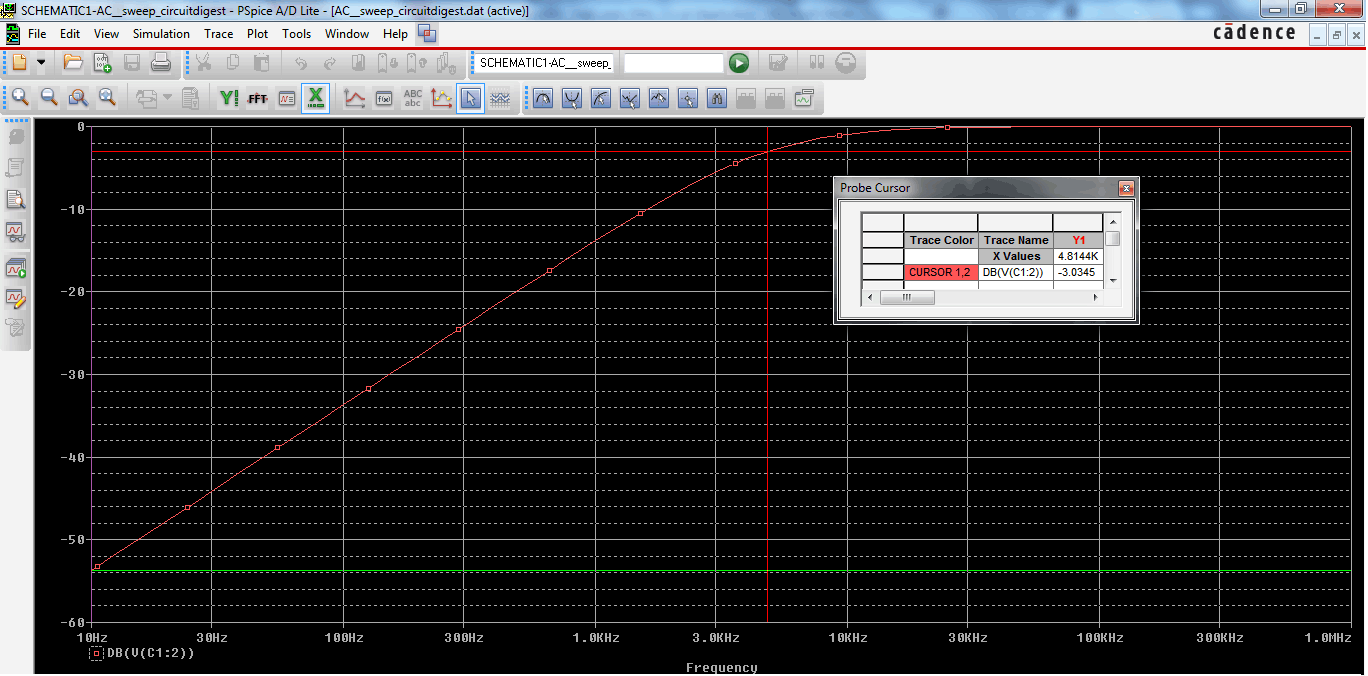As we can see the Frequency response (Also called as Bode Plot) we set the cursor at -3.03dB and get 4.814KHz Bandwidth Frequency.

### Phase Shift

Phase Angle denotes as φ (Phi) will be at the output is +45as of input signal, at -3dB or Cut-Off frequency.

As per the Frequency response of the filter shows us that it could pass all signals above cut-off frequency to the infinity. It is just theoretical practice. In practical and real world the passband is limited by the limitation of practical components.

The formula of Phase shift is not same as Low Pass filter as in low pass filter the phase became negative, but in high pass filter it is a positive phase shift, so the formula implies as:-

`Phase shift φ = arctan (1 / 2πfRC)`

Let’s see the phase shift curve of the circuit:-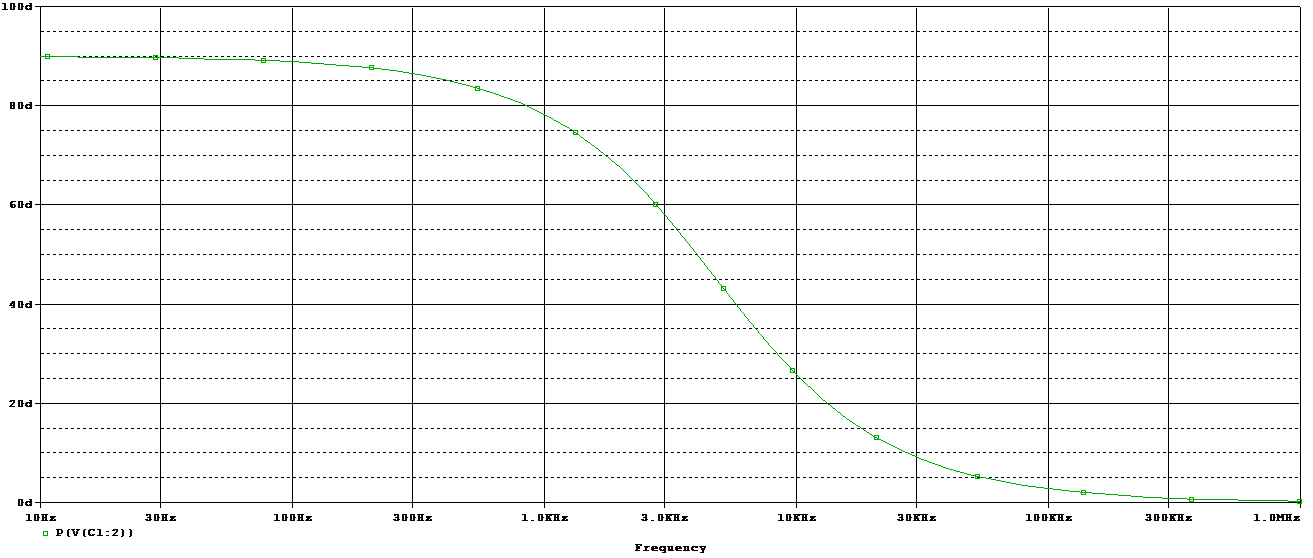This is the Phase shift of the circuit, used as practical example.

Let’s Find out the phase shift value at cut-off frequency:-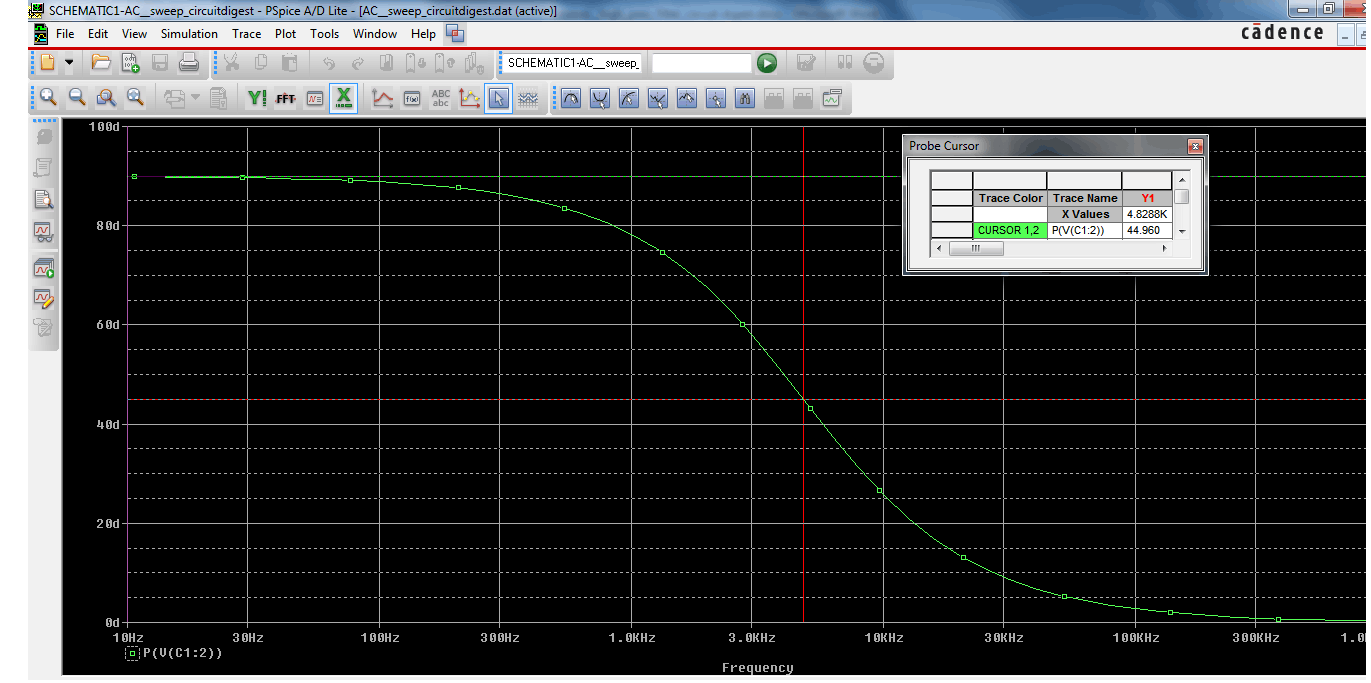We set the cursor at +45and get the result of the cut-off frequency 4.82KHz.

### Time constant

As we already learn before about phase shift and frequency response, the capacitor gets a charging and discharging effect from the input signal frequencies. This charging and discharging effect is Time Constant denotes as τ (Tau). It is also related with the cut-off frequency.

How?

`τ = RC = 1 / 2πfc`

Sometimes we need to know the cut-off frequency when we have the time constant value in such case altering the formula we can easily get that:-

```fc = 1 / 2πRC
Where RC = τ
fc = 1 / 2πτ```

Effect of Time Constant in High Pass Filter:

The High Pass filter is a Differentiator. If we feed Square Wave and the provide the it on a perfect time domain the output wave form of the filter produce spikes or short duration pulse. If the time constant is short durational the filter will produce differentiated square wave. We can differentiate a signal using this property of High Pass Filter.

### Second Order Low pass filter: Formulas, Calculations and Frequency Curves

When a two first order low pass RC stage circuit cascaded together it is called as second order filter as there are two RC stage networks.

Here is the circuit:-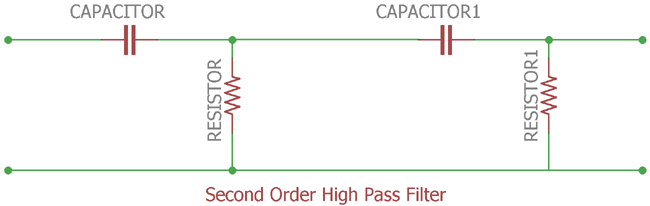This is a second order High Pass Filter. CAPACITOR and RESISTOR is the first order and CAPACITOR1 and RESISTOR1 is second order. Cascading together they form a second order High pass filter.

Second order filter has a role of slope of 2 x +20dB/decade or +40dB (12dB/octave).

Here is the response curve:-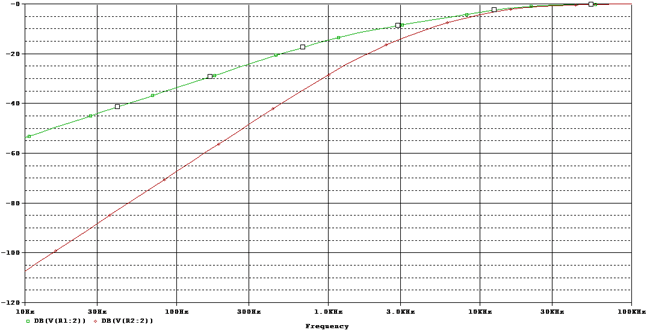The slope is +20dB/ Decade and the red one at the final output which has a slope of +40dB/Decade.

This will calculate the cut-off frequency of the second-order High pass circuit.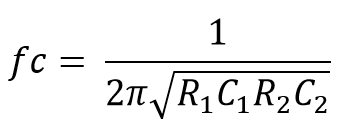Just as like Low Pass filter, it’s not so good to cascade two passive High Pass filters as dynamic impedance of each filter order effects other network in same circuitry.

### Applications

Low pass filter is widely used circuit in electronics.

Here are few applications:-